# [阅读型]深入理解C++多继承(multiple inheritance)与虚继承(virtual inheritance)的底层原理与实现-以g++为例

## 1. virtual function需要解决的问题

class A {
public:
virtual void f() {}
};
class B ： public A {
public:
virtual void f() {}
};

void func(A* p) {
p->f();
}


• 要执行的函数地址在哪里？C++要编译成汇编，具体为call addr，addr是一个内存地址不能是一个符号。
• 执行的这个函数的this指针是否正确？C++的类成员函数(non-static member function)执行是有一个隐藏的参数为this指针。可以理解为该成员函数的第一个参数是一个指针，指向这个对象的首地址。你可能想，this指针指向这个对象，它难道还会变化？很不幸，在多继承中this指针经常变化。

## 2. 单继承与vtable的出现

class A {
public:
virtual void f() {}
long int a;
};
class B : public A {
public:
virtual void f() {}
long int b;
};

class C : public B {
public:
virtual void f() {}
long int c;
};


### 对象内存布局

assert(sizeof(A) == 16);
assert(sizeof(B) == 24);
assert(sizeof(C) == 32);其中vtable ptr是一个指针指向.data.rel.rosection。这个section是只读区域，编译器专门在这个区域写上与继承相关的数据。vtable ptr A,B,C都是不相同的，编译器会为每一个类生成一个专门的vtable，vtable中存放着这个类中由virtual修饰的成员函数的地址。

### 回答两个关键的问题

• 要执行的函数地址在哪里？
ans: 在vtable中。程序会在运行时查找vtable，然后跳转到该函数。
//回到这个例子中，g++会怎么编译它?
void func(A* p) {
p->f();
}

//编译后的伪代码
void func(A* p) {
vtable*  vt = p->vtable;//vtbale就是p的首8字节，相当于 vt = ((void*)p);
Func f = vt; //f()是第一个virtual member function，所以vt的下标为0
f(p);//执行，p就是this，作为第一个参数
}


• 执行的这个函数的this指针是否正确？当然正确。有以下代码可以验证
C* c = new C();
B* b = c;//向上转型，安全
A* a = c; //向上转型，安全
assert(c == b);//true
assert(c == a);//true
//两个asser为true，这不显然的吗？
//可惜，在多继承中并不是这样


## 3. 多继承与thunk的出现

class A {
public:
virtual void f() {}
long int a;
};
class B : {
public:
virtual void f() {}
long int b;
};

class C : public A， public B {
public:
virtual void f() {}
long int c;
};


### 对象内存布局

assert(sizeof(A) == 16);
assert(sizeof(B) == 16);
assert(sizeof(C) == 40);//注意是5*8个字节当多继承出现的时候，从左到右按继承顺序，故在内存分析，依次是A、B、C，如上图所示。sizeof(C)==40是因为多出了sub-vtable ptr of B-in-C这8个字节。

direct base class order
When the direct base classes of a class are viewed as an ordered set, the order assumed is the order declared, left-to-right.

C* pc = new C();
B* pb = pc;                                  //向上转型，安全
A* pa = pc;                                  //向上转型，安全
cout << "pc == pb: " << (pc == pb) << endl;  // true, of course?
cout << "pc == pa: " << (pc == pa) << endl;  // true, of course?

cout << "(void*) pc == pb: " << (((void*)pc) == ((void*)pb))  // false, why?
<< endl;
cout << "(void*) pc == pa: " << (((void*)pc) == ((void*)pa))  // true, why?
<< endl;

//----------额外补充关于reinterpret_cast，行为又不一样，读者可以先忽略
cout << "----------------------reinterpret_cat\n";
pb = reinterpret_cast<B*>(pc);
pa = reinterpret_cast<A*>(pc);

cout << "pc == pb: " << (pc == pb) << endl;  // true
cout << "pc == pa: " << (pc == pa) << endl;  // false

cout << "(void*) pc == pb: " << (((void*)pc) == ((void*)pb))  // true
<< endl;
cout << "(void*) pc == pa: " << (((void*)pc) == ((void*)pa))  // true
<< endl;

//下面是一次实际运行的例子
C* c = new C();// c = 0x555555768e70; 堆上的地址
B* b = c;// b= 0x555555768e80; b=c+0x10;
A* a = c; // a = 0x555555768e70; a=c;


assert(c == b);//true
assert(((void*)c) == ((void*)b));//false, abort!
// c == 0x555555768e70; b == 0x555555768e80 == c + 0x10


• 多继承中，向上安全转型可能会导致this指针的值发生变化
• ==运算符在默认的情况下比较两个对象指针的时候，是比较这两个对象的地址，而不是比较这两个指针的值。

• 为什么this的值有时候必须发生变化
• 为什么在这个例子中是增加0x10sizeof(*a) == 16
sizeof(*b) == 16
sizeof(*c) == 40

### 回答两个关键的问题

• 要执行的函数地址在哪里？
//回到这个例子中，g++会怎么编译它?
void func(A* p) {
p->f();
}
void func(B* p) {
p->f();
}


• 执行的这个函数的this指针是否正确？不对，事情发生了变化。
//编译期间是不知道p到底是B本身，还是子类C
void func(B* p) {
p->f();
}

//编译后的伪代码
void func(B* p) {
vtable*  vt = p->vtable;//vtbale就是p的首8字节，相当于 vt = ((void*)p);
Func f = vt; //f()是第一个virtual member function，所以vt的下标为0

//编译期间是不知道p到底是B本身，还是子类C，这时候就出了问题
f(p);//如果是B本身
f(p-0x10);//如果是子类C，this需要修正
}


vtable ptr Bsub-vtable ptr B-in-C是不一样的，sub-vtable ptr B-in-C是是专门为class C中的B生成，和vtable类似，每一项存放者要call的函数地址。如果这个class B中的某个virtual函数被class C覆盖，则这一项会特别处理，称为thunk to C

0x555555554c21 <non-virtual thunk to C::f()>:	sub    rdi,0x10; rdi 是用于传递第一个参数,这一行修正了this
0x555555554c25 <non-virtual thunk to C::f()+4>:	jmp    0x555555554c16 <C::f()>

//编译后的伪代码
void func(B* p) {
vtable*  vt = p->vtable;
Func f = vt;
//如果*p是B本身，则f是B::f();
//如果*p是子类C，则f是non-virtual thunk to C::f();

f(p);
}


### 额外补充 关于vtable中的off-to-top

c++中实际上允许向下转型，虽然是不安全的，但是提供了dynamic_cast()

C* c = dynamic_cast<C*>(b);


dynamic_cast中是如何严格检查的？我这没有去扒源码，做一个猜测，知识来源一篇17年NDSS的paper2sub-vtable ptr B-in-C也就是下图的vtblptrC2的负1项存放着该对象的类型(RTTI)，负2项存放着off-to-top
Run-Time Type Information (RTTI). 可以通过typeid()提取出来，但是除了给标准库使用，不应该用于实际开发。
The Offset-to-Top field of the sub-vtables holds the value that has to be added to the thisptr to reach the beginning of the object.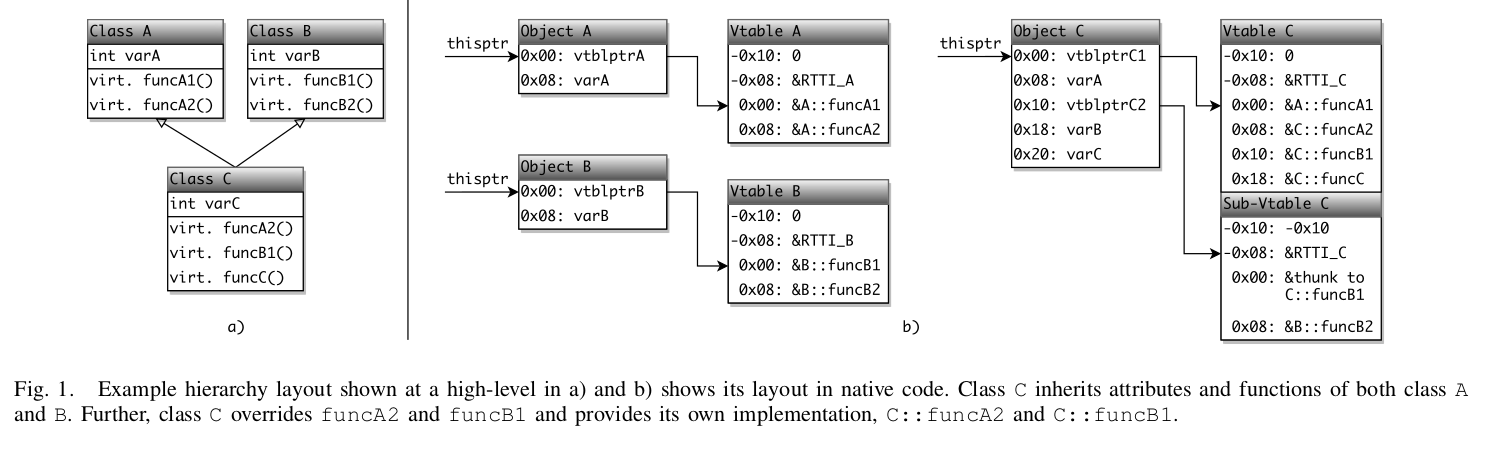## 4. 虚继承与VBO(vbase-offset)和VCO(vcall-offset)的出现

class A {
public:
virtual void f() {}
long int a;
};
class B : public A{
public:
virtual void f() {}
long int b;
};

class C : public A {
public:
virtual void f() {}
long int c;
};
class D : public B, public C {
public:
virtual void f() {}
long int c;
};


D d;
d.a = 1;//赋值. compile error, ambiguous
d.B::a = 1;//correct
d.C::a = 1;//correct


• Java. java中所有类都继承object，那不很容易菱形继承吗。好家伙，java不允许多继承，问题解决，完美。
• python. python是允许多继承的。python的成员方法和成员变量是类似dict的结构？有待研究python的内存模型。
• C++中为了解决这个问题，提出了虚继承。

### 对象内存布局

#define T long int
class A {
public:
virtual void af() {cout << "af" << endl;}
T a;
};

class B : public virtual A{
public:
virtual void bf() {cout << "bf" << endl;}
T b;
};

class C : public virtual A{
public:
virtual void cf() {cout << "cf" << endl;}
T c;
};

class D : public B, public C{
public:
virtual void df() {cout << "df" << endl;}
T d;
};



//于是有
sizeof(A) == 16;
sizeof(B) == 32;
sizeof(C) == 32;

sizeof(D) == 56;// 如果成员变量abcd是如下图的int类型，那么这里是48，因为c和d正好凑一起可以节约8个字节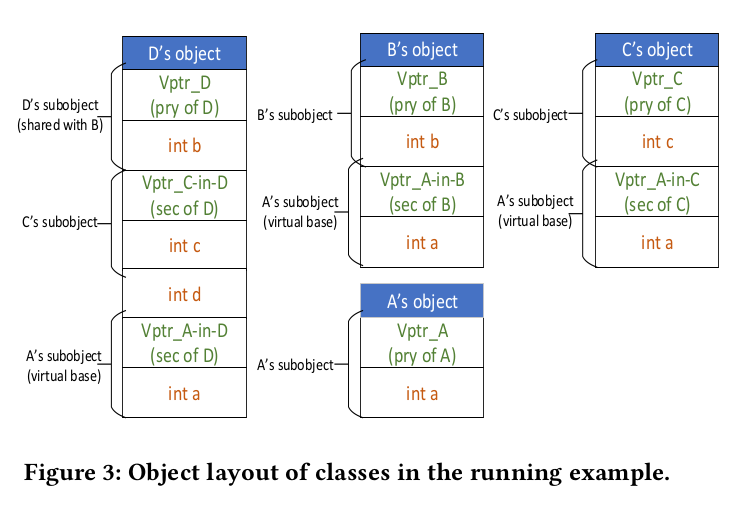class B: public A //普通继承A的时候

sizeof(B) == 24 // [0x00:Vptr], [0x08:a], [0x10:b]


class B: public virtual A //虚继承A的时候

sizeof(B) == 32 // [0x00:Vptr], [0x08:b], [0x10:Vptr_A-in-B], [0x18:a]


• 虚继承的class B的对象多出了Vptr_A-in-B
• 虚继承的class B的base class A的this是在对象的后面

不再满足

### 虚继承的取值困难

//考虑一个简单的取值例子
void f(B* p) {
int i = p->a;
}

//编译后的伪代码,出现矛盾。a的位置是编译时期不能确定的
void f(B* p) {
int i = ((long int*)p);//如果p指向的是B，p->a在第4的位置

int i = ((long int*)p);//如果p指向的是D，p->a在第7的位置
}


void f(B* p) {
A* b = p;//安全向上转型，但是this如何修正？
}


### vbase-offset的出现

vtable[-1]: RTTI (Run-Time Type Information)
vtable[-2]: OTT (off-to-top)
vtable[-3]: VBO (vbase-offset)

//于是
void f(B* p) {
int i = p->a;
}

//编译后的伪代码,矛盾解决。
void f(B* p) {
vtable* v = p->vtable;
long int vbo = v[-3];
A* a = (p+vbo); //找到了基类A的位置
int i = ((void*)a);// index 0是vtable， 1是long int a;
}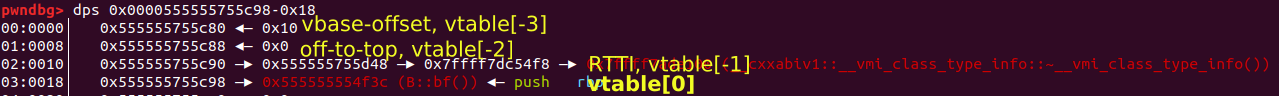下图是一篇paper3中完整的例子。CV(contruction vtable)与构造析构相关，先不考虑。图中每一列是这个类的vtable内容。如class D列，包含Vptr_D, Vptr_C-in-D, Vptr_A-in-D。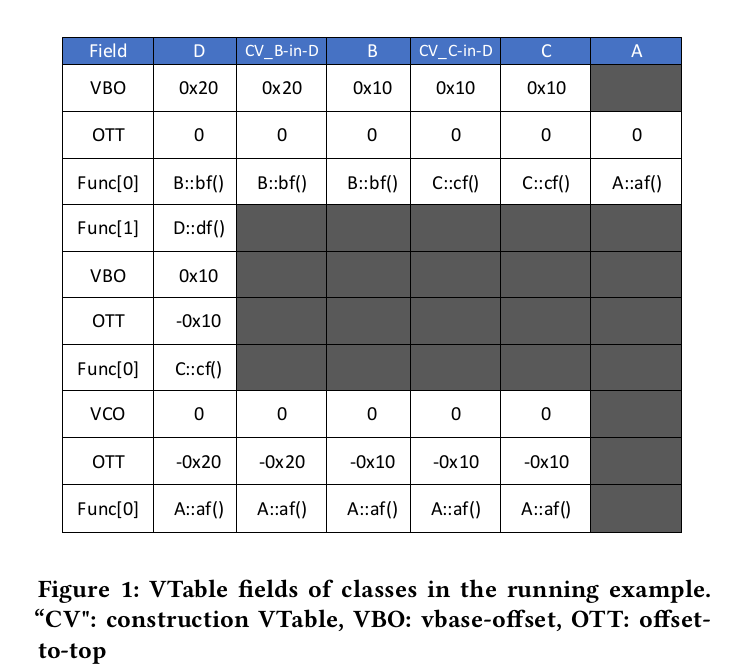### 回答两个关键的问题

• 要执行的函数地址在哪里？
和多继承一样，查表。vtable表的指针的地址固定存放在为this的首8字节。

• 执行的这个函数的this指针是否正确？
和多继承一样，由不同的表中的各个vritual fucntion的thunk承担修正this的职责。在虚继承中thunk修正出现了问题，thunk编译时不知道this的类型。所以，与vbase-offset相对应，每一个virtual function都需要vcall-offset。

//新的例子
#define T long int
class A {
public:
virtual void af() {cout << "af" << endl;}
T a;
};

class B : public virtual A{
public:
virtual void af() {cout << "B:af" << endl;}
virtual void bf() {cout << "bf" << endl;}
T b;
};

B* b = new B();
A* a = b; //向上转型，借助vbase-offset修正this
a->af(); //进入virtual thunk B:af(); 借助vcall-offset把this修正回来；实际效果是b->af();

   0x555555554d95 <virtual thunk to B::af()>:	mov    r10,QWORD PTR [rdi]
; r10 = vtable ptr

0x555555554d98 <virtual thunk to B::af()+3>:	add    rdi,QWORD PTR [r10-0x18]
; vcall-offset = r10[-3]; this = this + vcall-offset;

0x555555554d9c <virtual thunk to B::af()+7>:	jmp    0x555555554d5e <B::af()>



### 额外补充 vcall-offset的理解

#include <string>
#include <iostream>
using namespace std;
#define T long int
class A {
public:
virtual void af() {cout << "A:af" << endl;}
T a;
};

class B : public virtual A{
public:
virtual void af() {cout << "B:af" << endl;}
T b;
};

class C : public virtual A{
public:
T c;
};

class D : public B, public C{
public:
T d;
};

int main() {
A* a1 = new D();
a1->af();
// call rax<0x555555554f0d>(virtual thunk to B:af());
// vcall-offset = -40;

A* a2 = new B();
a2->af();
// call rax<0x555555554f0d>(virtual thunk to B:af());
// vcall-offset = -16;
return 0;
}


### 额外补充 虚继承向下强制转换

A* a = new D();
D* d = (D*)a; //compile error. 实际上还是能转换的
D* d = dynamic_cast<D*>(a);//correct，做了检查


## 5. 虚继承导致的vtable ptr初始化的困难与CV(Construction VTable)和VTT(Virtual Table Table)

• 执行构造函数，构造函数中需要对vtable初始化。编译器不知道要初始化的这个class B是在class D中的还是说就是原本的B。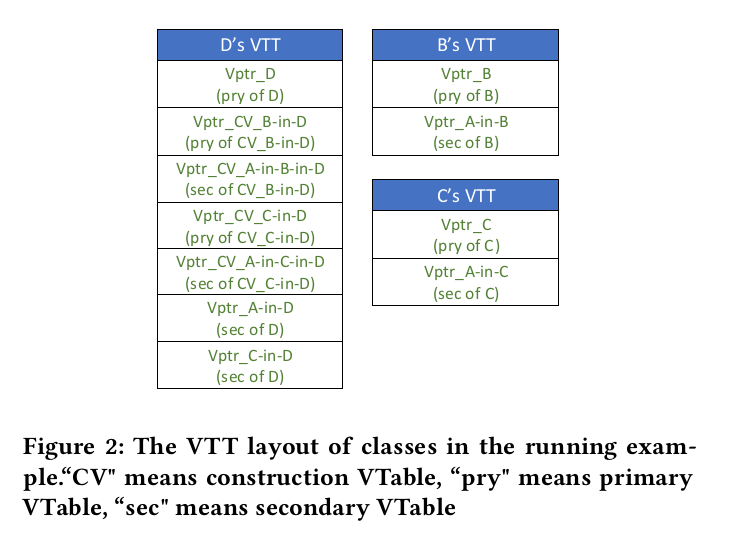1. Itanium C++ ABI (http://itanium-cxx-abi.github.io/cxx-abi/abi.html) ↩︎

2. Andre Pawlowski, Moritz Contag, Victor van der Veen, Chris Ouwehand, Thorsten Holz, Herbert Bos, Elias Athanasopoulos, and Cristiano Giuffrida. 2017. MARX : Uncovering Class Hierarchies in C++ Programs. In Proceedings of the 24th Annual Network and Distributed System Security Symposium. ↩︎ ↩︎ ↩︎

3. Erinfolami, Rukayat Ayomide, and Aravind Prakash. “Devil is Virtual: Reversing Virtual Inheritance in C++ Binaries.” Proceedings of the 2020 ACM SIGSAC Conference on Computer and Communications Security. 2020. ↩︎ ↩︎ ↩︎ ↩︎被折叠的  条评论 为什么被折叠?到【灌水乐园】发言easylyou

¥1 ¥2 ¥4 ¥6 ¥10 ¥20获取中扫码支付点击重新获取扫码支付1.余额是钱包充值的虚拟货币，按照1:1的比例进行支付金额的抵扣。
2.余额无法直接购买下载，可以购买VIP、付费专栏及课程。余额充值